GED Math : Area of a Quadrilateral

Example Questions

1 2 3 4 6 Next →

Example Question #51 : Area Of A Quadrilateral

A square and a circle share a center as shown by the figure below.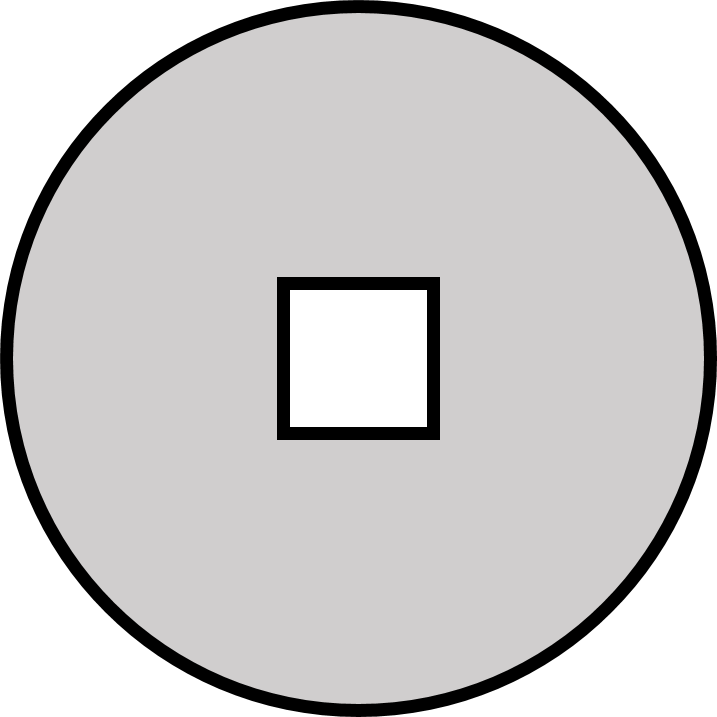If the length of a side of the square isand is half the length of the radius of the circle, to the nearest hundredths place, find the area of the shaded region.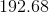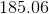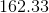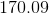Explanation:

In order to find the area of the shaded region, we will need to find the area of the circle then subtract the area of the square from it.

Start by finding the area of the square. Since we know the side length of the square, we can write the following: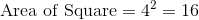Next, we find the area of the circle. Since the question states that the length of a side of the square is half the radius of the circle, the radius of the circle must be. Thus, we can find the area of the circle.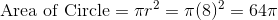Now, subtract these two values to find the area of the shaded region.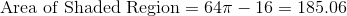Example Question #52 : Area Of A Quadrilateral

If the area of square is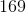, what is its perimeter?Explanation:

Figuring out the perimeter of a square from this information is luckily pretty easy. Since the sides of a square are all the same size, you know also that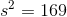.

Taking the square root of both sides, you get: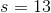Now, the perimeter of the square is just, or.

Example Question #53 : Area Of A Quadrilateral

If the perimeter of a square is, what is its area?Explanation:

Since the four sides of a square are equal, you know that the perimeter of a square is defined as: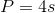For our data, this is: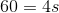Solving for, you get: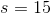Now, the area of a square is just: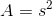or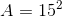, which is the same as.

1 2 3 4 6 Next →